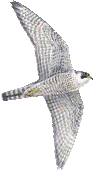## g_anaeig

VERSION 4.6.5
Mon 2 Dec 2013

### Description

g_anaeig analyzes eigenvectors. The eigenvectors can be of a covariance matrix (g_covar) or of a Normal Modes analysis (g_nmeig).

When a trajectory is projected on eigenvectors, all structures are fitted to the structure in the eigenvector file, if present, otherwise to the structure in the structure file. When no run input file is supplied, periodicity will not be taken into account. Most analyses are performed on eigenvectors -first to -last, but when -first is set to -1 you will be prompted for a selection.

-comp: plot the vector components per atom of eigenvectors -first to -last.

-rmsf: plot the RMS fluctuation per atom of eigenvectors -first to -last (requires -eig).

-proj: calculate projections of a trajectory on eigenvectors -first to -last. The projections of a trajectory on the eigenvectors of its covariance matrix are called principal components (pc's). It is often useful to check the cosine content of the pc's, since the pc's of random diffusion are cosines with the number of periods equal to half the pc index. The cosine content of the pc's can be calculated with the program g_analyze.

-2d: calculate a 2d projection of a trajectory on eigenvectors -first and -last.

-3d: calculate a 3d projection of a trajectory on the first three selected eigenvectors.

-filt: filter the trajectory to show only the motion along eigenvectors -first to -last.

-extr: calculate the two extreme projections along a trajectory on the average structure and interpolate -nframes frames between them, or set your own extremes with -max. The eigenvector -first will be written unless -first and -last have been set explicitly, in which case all eigenvectors will be written to separate files. Chain identifiers will be added when writing a .pdb file with two or three structures (you can use rasmol -nmrpdb to view such a .pdb file).

Overlap calculations between covariance analysis:
Note: the analysis should use the same fitting structure

-over: calculate the subspace overlap of the eigenvectors in file -v2 with eigenvectors -first to -last in file -v.

-inpr: calculate a matrix of inner-products between eigenvectors in files -v and -v2. All eigenvectors of both files will be used unless -first and -last have been set explicitly.

When -v, -eig, -v2 and -eig2 are given, a single number for the overlap between the covariance matrices is generated. The formulas are:
difference = sqrt(tr((sqrt(M1) - sqrt(M2))^2))
normalized overlap = 1 - difference/sqrt(tr(M1) + tr(M2))
shape overlap = 1 - sqrt(tr((sqrt(M1/tr(M1)) - sqrt(M2/tr(M2)))^2))
where M1 and M2 are the two covariance matrices and tr is the trace of a matrix. The numbers are proportional to the overlap of the square root of the fluctuations. The normalized overlap is the most useful number, it is 1 for identical matrices and 0 when the sampled subspaces are orthogonal.

When the -entropy flag is given an entropy estimate will be computed based on the Quasiharmonic approach and based on Schlitter's formula.

### Files

optionfilenametypedescription
-v eigenvec.trr Input Full precision trajectory: trr trj cpt
-v2 eigenvec2.trr Input, Opt. Full precision trajectory: trr trj cpt
-f traj.xtc Input, Opt. Trajectory: xtc trr trj gro g96 pdb cpt
-s topol.tpr Input, Opt. Structure+mass(db): tpr tpb tpa gro g96 pdb
-n index.ndx Input, Opt. Index file
-eig eigenval.xvg Input, Opt. xvgr/xmgr file
-eig2 eigenval2.xvg Input, Opt. xvgr/xmgr file
-comp eigcomp.xvg Output, Opt. xvgr/xmgr file
-rmsf eigrmsf.xvg Output, Opt. xvgr/xmgr file
-proj proj.xvg Output, Opt. xvgr/xmgr file
-2d 2dproj.xvg Output, Opt. xvgr/xmgr file
-3d 3dproj.pdb Output, Opt. Structure file: gro g96 pdb etc.
-filt filtered.xtc Output, Opt. Trajectory: xtc trr trj gro g96 pdb cpt
-extr extreme.pdb Output, Opt. Trajectory: xtc trr trj gro g96 pdb cpt
-over overlap.xvg Output, Opt. xvgr/xmgr file
-inpr inprod.xpm Output, Opt. X PixMap compatible matrix file

### Other options

optiontypedefaultdescription
-[no]h bool no Print help info and quit
-[no]version bool no Print version info and quit
-nice int 19 Set the nicelevel
-b time 0 First frame (ps) to read from trajectory
-e time 0 Last frame (ps) to read from trajectory
-dt time 0 Only use frame when t MOD dt = first time (ps)
-tu enum ps Time unit: fs, ps, ns, us, ms or s
-[no]w bool no View output .xvg, .xpm, .eps and .pdb files
-xvg enum xmgrace xvg plot formatting: xmgrace, xmgr or none
-first int 1 First eigenvector for analysis (-1 is select)
-last int -1 Last eigenvector for analysis (-1 is till the last)
-skip int 1 Only analyse every nr-th frame
-max real 0 Maximum for projection of the eigenvector on the average structure, max=0 gives the extremes
-nframes int 2 Number of frames for the extremes output
-[no]split bool no Split eigenvector projections where time is zero
-[no]entropy bool no Compute entropy according to the Quasiharmonic formula or Schlitter's method.
-temp real 298.15 Temperature for entropy calculations
-nevskip int 6 Number of eigenvalues to skip when computing the entropy due to the quasi harmonic approximation. When you do a rotational and/or translational fit prior to the covariance analysis, you get 3 or 6 eigenvalues that are very close to zero, and which should not be taken into account when computing the entropy.